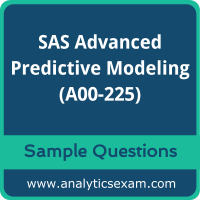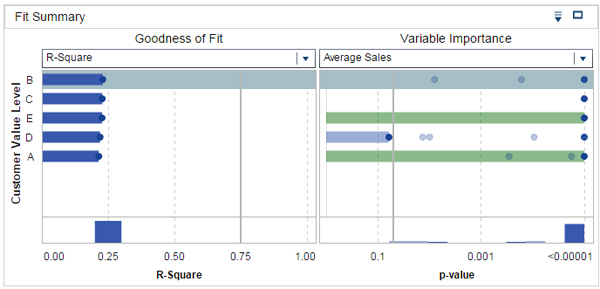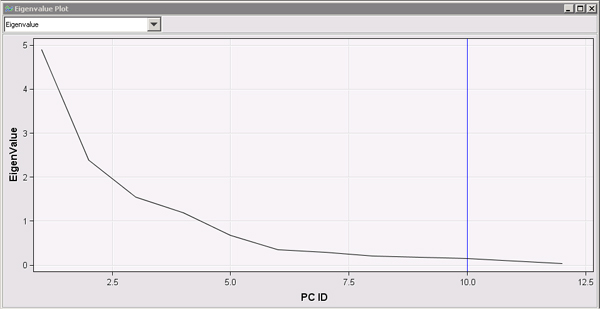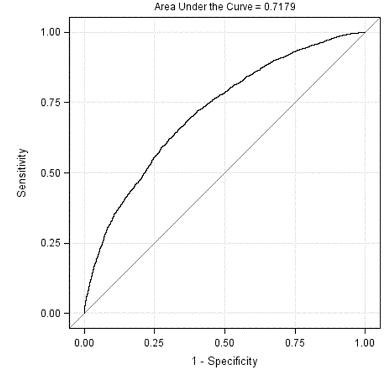# SAS Advanced Analytics Professional (A00-225) Certification Exam Sample QuestionsHere are the sample questions which will help you be familiar with SAS Advanced Predictive Modeling (A00-225) exam style and structure. We encourage you to try our Demo SAS Advanced Analytics Professional Certification Practice Exam to measure your understanding of exam structure in an environment which simulates the SAS Certified Advanced Analytics Professional Using SAS 9 Certification test environment.

To make your preparation more easy for SAS Certified Advanced Analytics Professional Using SAS 9 (A00-225) exam, we strongly recommend you to use our Premium SAS Advanced Analytics Professional Certification Practice Exam. According to our survey with certified candidates, you can easily score 85% in your actual SAS Certification exam if you can score 100% in our premium SAS Advanced Analytics Professional Certification practice exams.

## SAS A00-225 Sample Questions:

01. What is a linear Perceptron?
a) A linear Perceptron is a general linear model.
b) A linear Perceptron is a generalized linear model.
c) A linear Perceptron is a non-parametric model.
d) A linear Perceptron is a nonlinear model.

02. Consider a Generalized Additive Neural Network (GANN) with 3 continuous inputs and 2 hidden nodes for each input. How many parameters do you need to estimate when training the neural network?
a) 19
b) 21
c) 22
d) 25

03. Refer to the fit summary from SAS Visual Statistics in the exhibit below.What can be concluded from the fit summary?
a) Customer Value Level is not a significant predictor in this model.
b) Customer Value Level C has no important variables associated with it.
c) Average Sales is a significant predictor when Customer Value Level = E.
d) Average Sales is an important predictor when Customer Value Level = C.

04. When mean imputation is performed on data after the data is partitioned for honest assessment, what is the most appropriate method for handling the mean imputation?
a) The sample means from the validation data set are applied to the training and test data sets.
b) The sample means from the training data set are applied to the validation and test data sets.
c) The sample means from the test data set are applied to the training and validation data sets.
d) The sample means from each partition of the data are applied to their own partition.

05. Which software does the SAS Enterprise Miner Open Source Integration node use to execute R programs?
a) SAS/IML
b) SAS/STAT
c) SAS/ACCESS
d) SAS/OR

06. Refer to the Eigenvalue plot from SAS Enterprise Miner shown below.According to the Kaiser-Guttman method, how many principal components should be retained?
a) 6
b) 4
c) 10
d) 1

07. Which statement is true for negative binomial and Poisson regression models?
a) Poisson regression models are used for count data, and negative binomial models are used for binary responses.
b) The canonical link function for Poisson regression is the log, while for negative binomial it is the logit.
c) Negative binomial models accommodate negative integers while Poisson regression does not.
d) Poisson regression is a special case of negative binomial regression.

08. A predictive model uses a data set that has several variables with missing values. What two problems can arise with this model?
(Choose two.)
a) The model will likely be overfit.
b) There will be a high rate of collinearity among input variables.
c) Fewer observations will be used in the model building process.
d) New cases with missing values on input variables cannot be scored without extra data processing.

09. Refer to the exhibit:For the ROC curve shown, what is the meaning of the area under the curve?
a) percent concordant plus percent tied
b) percent concordant plus (.5 * percent tied)
c) percent concordant plus (.5 * percent discordant)
d) percent discordant plus percent tied

10. What is the maximum number of response variables that SAS Visual Statistics allows for a decision tree?
a) 1
b) 2
c) 3
d) 4